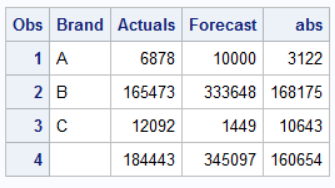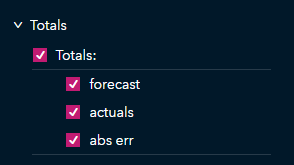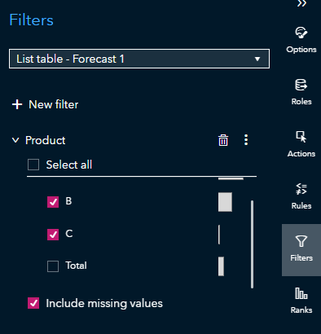## Incorrect aggregation of ABS values

Hi all,

I am trying to calculate a forecast accuracy in SAS VA 8.5.2 and the absolute error is not correctly aggregating/summing.

Here is a table similar to the one I am working with:

 Brand Actuals Forecast ABS Error (Abs(Actuals-Forecast) A 6,878 10,000 3,122 B 165,473 333,648 168,175 C 12,092 1,449 10,643 Total 184,443 345,097 160,654

The ABS Error is correct, but the total is incorrect. It is not recognizing that these values are positive. The total should be 181,940.

Due to this incorrect aggregation, my forecast accuracy calculation is coming out to an unexpected and incorrect value and I'm wondering how I can correct this.

1 ACCEPTED SOLUTION

Accepted Solutions

## Re: Incorrect aggregation of ABS values

Hi all!

I figured it out.

When creating a new calculated value for the abs(error) make sure to change the result type fromtoon the top right

Calculated fields switch to aggregated measure once you involve sum, but if you try to change it back, it errors out. So make sure to keep values as numeric so that each observation is calculated correctly (this will seem to be the case using either method, but where it falls apart is when you aggregate in visuals or total-up in a list table).

6 REPLIES 6

## Re: Incorrect aggregation of ABS values

Sounds like you have the order operations wrongs.

You need to calculate ABS(actuals - forecast) first.

Then generate the bottom line as the sums of those three variables.

It looks instead you generated the sums first and then did the same abs(actuals - forecast) for the summary line as you did for the detail lines.

## Re: Incorrect aggregation of ABS values

Hi Tom,

I'm sorry, I'm not following.

So right now I have 400,000 (ignore previous values) when I total absolute error value. This is calculated by

Abs(forecast - actuals)

But when summing up via list table total, I get a far lower number. Something around 180,000.

## Re: Incorrect aggregation of ABS values

You have to figure out how to get VA to behave like normal analysis.

``````data have;
input Brand \$ Actuals Forecast;
cards;
A 6878 10000
B 165473 333648
C 12092 1449
;

data step1;
set have;
abs = abs(actuals - forecast);
run;

proc print;
id brand;
sum Actuals Forecast abs ;
run;``````You either loaded that extra TOTAL row with the real data.  Or you had it first calculate the totals and then asked it to take the difference of the totals.  Like this:

``````proc summary data=have;
var actuals forecast ;
output out=totals(drop=_:) sum=;
run;

data step1;
set have totals;
abs = abs(actuals-forecast);
run;

proc print;
run;
``````You are doing the steps out of order.

## Re: Incorrect aggregation of ABS values

```abs('forecast'n - 'actuals'n)
```

Then you should be good.

Based on what you have, it looks like Total is a part of your list table, which is why it's aggregating that way; however, this is the correct calculation for your overall forecast. Consider the following hypothetical forecast where the Total row is the sum of each column:

 Product Forecast Actual Error Abs Error A 0 100 -100 100 B 300 200 100 100 C 300 300 0 0 Total 600 600 0 200

Total is a bottom-up forecast created by summing up products A, B, and C. The individual forecasts of A and B are off by -100 and 100 respectively, but the overall bottom-up forecast has an error of 0: the errors in products A and B cancel each other out to create a perfect overall forecast. If you're trying to figure out how good your individual forecasts are, this can be a deceptive metric if your individual forecasts aren't great. The sum of the absolute errors tells us there are 200 total errors among all of the individual forecasts, but it does not mean that the overall forecast has an absolute error of 200.

If you want to capture the total number of absolute errors of all forecasts, you need to remove the Total row and have Visual Analytics calculate the sum for you with the Totals option in a list table:You can filter out your Total row with an object filter so you do not need to reload the data:However, summing up all of the absolute errors is a relative measure and doesn't necessarily tell the whole story. One forecast could have a lot of errors while other forecasts could have a few. It's one indicator that there may be a problem with one or more forecasts, but it cannot be used to judge the accuracy of all hierarchical forecasts, especially if one hierarchy has large values while another hierarchy has low values. If you would like some good tips on how to judge the average accuracy of your hierarchical forecasts, I would recommend posting in the Forecasting and Econometrics forum.

Generally I have always looked at MASE or MAPE values and sorted them in ascending order to view how well individual forecasts are doing.

## Re: Incorrect aggregation of ABS values

Thanks Stu!

My actual formula in VA looks like so:

`Abs(Sum [_ByGroup_] (( 'Actuals'n - 'Forecast'n )))`

does this change anything? Because total is not actually part of my table, but the output of VA when adding total for a list view. I only have it that way for the time being to troubleshoot why my forecast isn't coming out as expected.

And I understand why this can be deceptive or maybe not best practice, but I am also layering other views where a traditional MAPE is shown (no abs errorin calc) and even a SMAPE value. We just deemed the absolute forecast as the best way to show this forecast for a particular hierarchy to satisfy a particular vendor's SLA requirement.

## Re: Incorrect aggregation of ABS values

Hi all!

I figured it out.

When creating a new calculated value for the abs(error) make sure to change the result type fromtoon the top right

Calculated fields switch to aggregated measure once you involve sum, but if you try to change it back, it errors out. So make sure to keep values as numeric so that each observation is calculated correctly (this will seem to be the case using either method, but where it falls apart is when you aggregate in visuals or total-up in a list table).

Discussion stats
• 6 replies
• 326 views
• 0 likes
• 3 in conversation